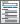Home  /  Products  /  Features  /  Resampling and simulation methods

## Resampling and simulation methods

Random-number functions• Uniform over interval (0,1)
• Uniform over interval (a,b)
• Uniform integers over interval [a,b]
• Standard normal
• Normal, mean m and std. deviation 1
• Normal, mean m and std. deviation s
• Beta
• Binomial
• Cauchy
• Chi-squared
• Exponential
• Gamma
• Hypergeometric
• Inverse Gaussian
• Laplace
• Logistic, mean m and std. deviation π/√3
• Logistic, mean 0, scale s, std. deviation sπ/√3
• Logistic, mean m, scale s, std. deviation sπ/√3
• Negative binomial
• Poisson
• Student's t
• Weibull, shape a, scale b
• Weibull, shape a, scale b, location g
• Weibull (proportional hazards), shape a, scale b
• Weibull (proportional hazards), shape a, scale b, location g
• 64-bit Mersenne Twister generator
• Stream version of 64-bit Mersenne Twister• Allow random numbers to be drawn correctly on multiple machines or in multiple processes
• Each stream is independent of other streams
• When the stream generator is used, all random-number functions automatically become stream enabled.
• 32-bit KISS generator

Jackknife estimationMonte Carlo simulation• Check the performance of statistical methods
• Simulate Stata commands
• Simulate community-contributed commands

Permutation tests